2021/10/13

# 程序设计基本元素

John Locke 在 1690 年《有关人类理解的随笔》中写到：心智的活动，除了产生各种简单的认识外，还通过组合的方式形成复合认识，此外还可以将两个简单或复合认识对照以获得相对关系，将有关认识和所有其它认识隔离开，就是抽象，所有具有普遍性的认识都是这样得到的。

``````(+ (* 3
(+ (* 2 4)
(+ 3 5)
(+ (- 10 7)
6))
``````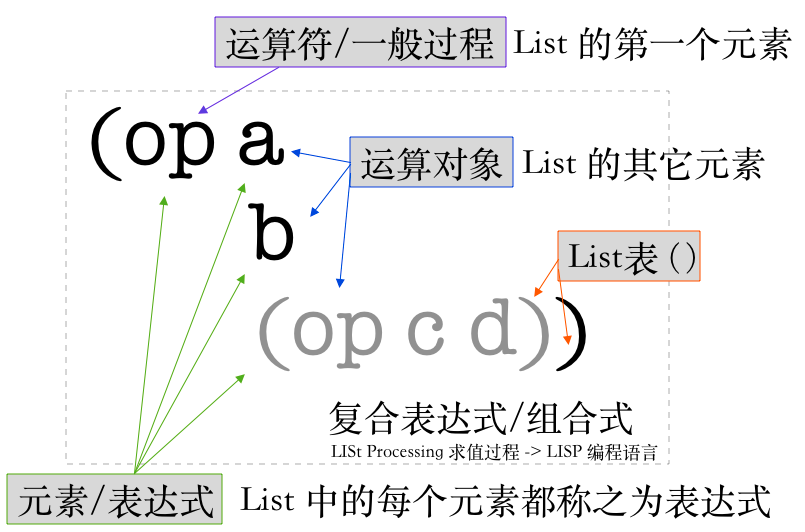``````(define (square x) (* x x))
(define (sum-of-square x y) (+ (square x) (square y)))
(define (f a) (sum-of-square (+ a 1) (* a 2)))
``````

``````(define (gcd a b) (if (= b 0) a (gcd b (remainder a b))))

(gcd 206 40)
(gcd 40 (R 206 40))
(gcd 6 (R 40 6))
(gcd 4 (R 6 4))
(gcd 2 (R 2 2))

(gcd 206 40)
(gcd 40 (R 206 40))
(gcd (R 206 40) (R 40 (R 206 40)))
(gcd (R 40 (R 206 40)) (R (R 206 40) (R 40 (R 206 40))))
(gcd (R (R 206 40) (R 40 (R 206 40))) (R (R 40 (R 206 40)) (R (R 206 40) (R 40 (R 206 40))))
``````

``````(define (>= x y) (not (< x y))
(define hour 13)
(define say-hello (if (> hour 11) "morning" "noon"))
(define to-do (cond ((and (> hour 6) (< hour 18)) "work") (else "sleep"))
``````

``````;应用序问题
(define (if-2 pre-c then-c else-c)
(cond (pre-c then-c) (else else-c)))
(display "\n")
(display (if-2 (> 2 1) 10 20))
``````

``````;是什么和怎么做的区别，一个实现求平方的复合过程
(define (sqrt-original x g)
(if (< (/ (if (< (- x (* g g)) 0)
(- (- x (* g g)))
(- x (* g g)))
x)
0.01)
g
(sqrt-original
x (/ (+ (/ x g) g) 2))))
``````

``````;过程抽象的内涵：捕获变量（形参）和自由变量（外部符号）
(display "\nFor Sqrt: \n")
(define (abs x) (if (< x 0) (- x) x))
(define (is-good-sqrt-1)
(< (abs (- x (* g g))) 0.01))
(define (is-good-sqrt-2 x g)
(< (/ (abs (- x (* g g))) x) 0.01))
(define (improve-sqrt x g)
(guess-sqrt x (/ (+ (/ x g) g) 2)))
(define (guess-sqrt x g)
(if (is-good-sqrt-2 x g) g
(improve-sqrt x g)))
(define (sqrt x) (guess-sqrt x 1.0))
(display (sqrt 10))
``````

``````;块结构：嵌套的定义，更清晰的结构
(printf "\nFor Cube: \n")
(define (cube x)
(define (is-good-cube x g)
(< (/ (abs (- x (* g g g))) x) 0.01))
(define (improve-cube x g)
(guess-cube x (/ (+ (/ x (* g g))
(* g 2)) 3)))
(define (guess-cube x g)
(if (is-good-cube x g) g
(improve-cube x g)))
(guess-cube x 1.0))
(display (cube 10))
``````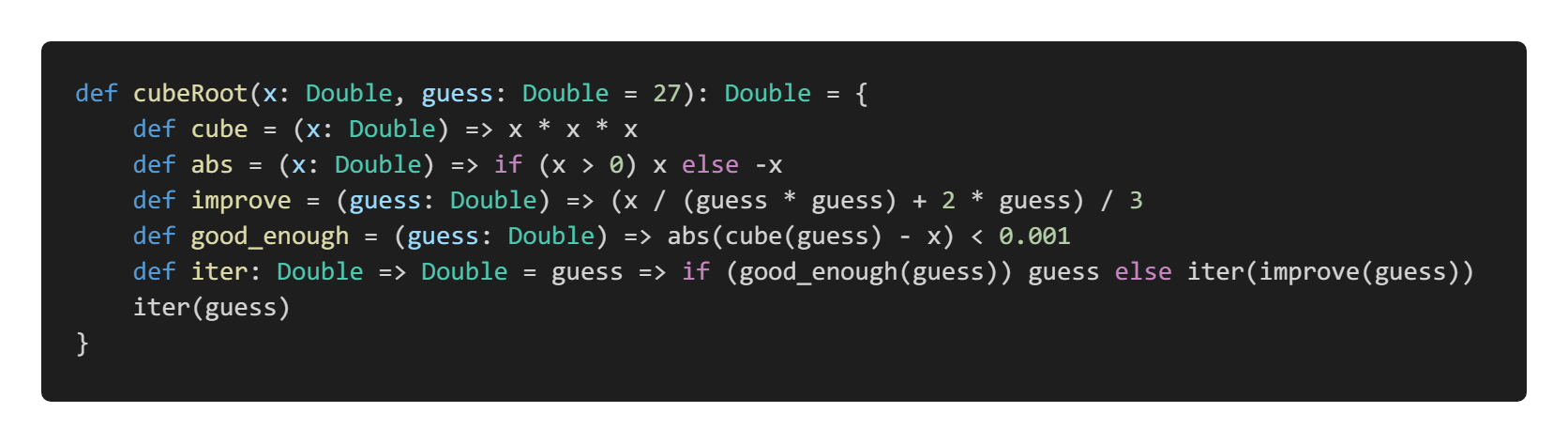# 过程所产生的计算

``````(define (factorial n)
;计算阶乘 n x (n-1) x (n-2) .. x 1，递归方式
(if (= n 1) 1
(* n (factorial (- n 1)))))
(define (factorial2 n)
;计算阶乘 1 x 2 x .. x (n-1) x n，迭代方式
(define (fact-iter now)
(if (= now n)
n
(* now (fact-iter (+ now 1)))))
(fact-iter 1))
(printf "fac:~s, fac2:~s" (factorial 10) (factorial2 10))
``````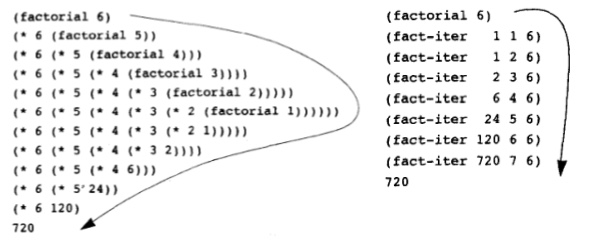``````(define (fib n)
;树状递归的例子，大量的冗余计算出现
(cond ((= n 0) 0)
((= n 1) 1)
(else (+ (fib (- n 2)) (fib (- n 1))))))
(define (fib2 n)
;尾递归优化：且更改算法以避免树状冗余分支
(define (iter a b count)
(if (= count 0)
b
(iter (+ a b) a (- count 1))))
(iter 1 0 n))
``````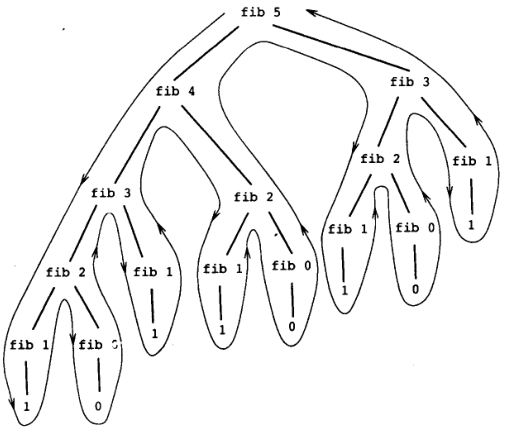``````(define (count-change amount)
(define (first-denomination kinds-of-coins)
;根据可用的硬币总数返回第一种硬币的币值
(cond ((= kinds-of-coins 1) 1)
((= kinds-of-coins 2) 5)
((= kinds-of-coins 3) 10)
((= kinds-of-coins 4) 25)
((= kinds-of-coins 5) 50)))
(define (cc amount kinds-of-coins)
(cond ((= amount 0) 1)
((or (< amount 0) (= kinds-of-coins 0)) 0)
(else (+ (cc amount (- kinds-of-coins 1))
(cc (- amount (first-denomination kinds-of-coins))
kinds-of-coins)))))
(cc amount 5))
(printf "kind of change ~s" (count-change 100))
``````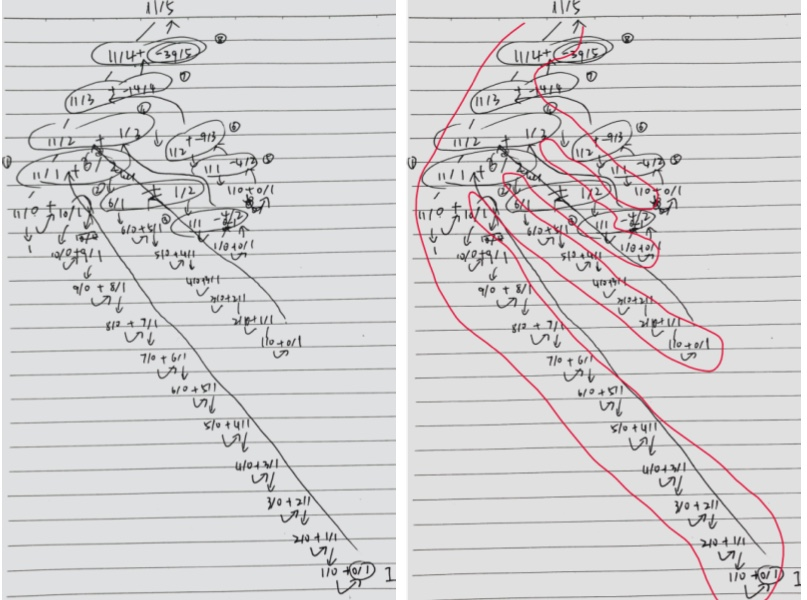``````(define (f n)
(if (< n 3)
n
(+ (f (- n 1))
(* 2 (f (- n 2)))
(* 3 (f (- n 3))))))
(define (f2 n)
(define (fa fnm3 fnm2 fnm1 mark)
(define fn (+ fnm1 (* 2 fnm2) (* 3 fnm3)))
(cond ((= mark n) fn)
(else (fa fnm2 fnm1 fn (+ mark 1)))))
(if (< n 3) n (fa 0 1 2 3)))
``````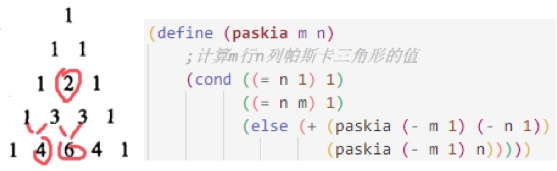``````(define (sine angle)
;当角 x 足够小，sinx ≈ x，反之可通过 sinx = 3sin(x/3) - 4sin^3(x/3) 减小其参数大小
(define (cube x) (* x x x))
(define (p x)
(- (* 3 x) (* 4 (cube x))))
(if (not (> (abs angle) 0.1))
angle
(p (sine (/ angle 3.0)))))
(printf "sine 12.15 is ~d" (sine 12.15))
``````

``````(define (expt1 b n)
;递归实现 θ(n) step and space
(if (= n 0) 1 (* b (expt1 b (- n 1)))))
(define (expt2 b n)
;迭代实现 θ(n) step, θ(1) space
(define (exp-inner m now)
(if (< m n) (exp-inner (+ m 1) (* now b)) now))
(exp-inner 0 1))
(define (expt3 b n)
;特殊递归实现 θ(logn) step and space
(define (square x) (* x x))
(define (even? n) (= (remainder n 2) 0))
(cond ((= n 0) 1)
((even? n) (square (expt3 b (/ n 2))))
(else (* b (expt3 b (- n 1))))))
(define (expt4 b n)
;特殊迭代实现 θ(logn) step and space
(define (even? n) (= (remainder n 2) 0))
(define (exp-inner a n)
(cond ((< n 2) a)
((even? n) (exp-inner (* a (* b b)) (/ n 2)))
(else (exp-inner (* a b) (- n 1)))))
(if (= n 1) b (exp-inner 1 n)))
``````

``````(define (multi a b)
;乘法的基本递归形式：每次 b 下降 1，a 增加 1 倍，θ(n) steps
(if (= b 0) 0 (+ a (* a (- b 1)))))
(define (multi2 a b)
;乘法的高效递归形式：每次 b 下降一半，a 增加 2 倍，θ(logn) steps
(define (double x) (+ x x))
(define (halve x) (/ x 2))
(define (even? n) (= (remainder n 2) 0))
(cond ((= b 0) 0)
((even? b)
(double (multi2 a (halve b))))
(else (+ a (multi2 a (- b 1))))))
(define (multi3 a b)
;乘法的高效迭代形式：θ(logn) steps
(define (double x) (+ x x))
(define (halve x) (/ x 2))
(define (even? n) (= (remainder n 2) 0))
(define (multi-inner now-b now-a)
(cond ((< now-b 2) now-a)
((even? now-b) (multi-inner (halve now-b) (double now-a)))
(else (multi-inner (- now-b 1) (+ now-a now-b)))))
(multi-inner b a))
``````

``````(define (fib n)
;树状递归的例子，大量的冗余计算出现
(cond ((= n 0) 0)
((= n 1) 1)
(else (+ (fib (- n 2)) (fib (- n 1))))))
(define (fib2 n)
;尾递归优化：且更改算法以避免树状冗余分支
(define (iter a b count)
(if (= count 0)
b
(iter (+ a b) a (- count 1))))
(iter 1 0 n))
(define (fib3 n)
;斐波那契数列的线性迭代解决方法 2
;这种方法 a<-a+b, b<-a 称之为 T 变换, 将初始值为 n 的 count 的下降作为状态
;容易发现 T 变换 n 此将导致 Fib(n+1) 和 Fib(n)
(define (f-iter a b count)
(if (= count 0) b (f-iter (+ a b) a (- count 1))))
(f-iter 1 0 n))
(define (fib4 n)
;斐波那契数列的对数迭代解决方法
;在 f3 中的 T 变换本质上是 Tpq 变换族中 p=0,q=1 对于对偶 (0,1) 进行变换的特例。
;即 Tpq 是对于对偶 (a,b) 按照 a<-bq+aq+ap 和 b<-bp+aq 规则进行的变换。因为 Tpq 变换
;两次等价于一种特殊的 p q 值的应用，因此可以连续求平方的方式计算 Tn。
;计算过程非常简单，用 a1,b1,p,q 表示 a2,b2，用 a2,b2,p,q 表示 a3,b3
;然后将表示 a3,b3 的 a2,b2 代换为 a1,b1, 得到 a3,b3 和 a1,b1,p,q 的表示关系，最后
;对照 a3,b3 和 a1,b1,p',q' 的关系，即可得到 p-p' 以及 q-q' 的关系。
(define (even? n) (= (remainder n 2) 0))
(define (f-iter a b p q count)
(cond ((= count 0) b)
((even? count)
(f-iter a b (+ (* p p) (* q q)) (+ (* q q) (* 2 p q)) (/ count 2)))
(else (f-iter (+ (* b q) (* a q) (* a p))
(+ (* b p) (* a q))
p q (- count 1)))))
(f-iter 1 0 0 1 n))
``````

``````(define (prime? n)
;一种直接寻找素数的方法：从 2 开始去除，直到 √n 为止，
;原因很简单，如果 d 是 n 的因子，则 d/n 也是，d 和 d/n 都不会大于 √n
;2 - √n 返回内找不到则返回 n 表示为素数。有 θ(√n) 的增长阶
(define (square x) (* x x))
(define (divides? a b) (= (remainder b a) 0))
(define (find-divisor n test)
(cond ((> (square test) n) n)
((divides? test n) test)
(else (find-divisor n (next test)))))
;如果不能被 2 整除，那么后续所有偶数都不需要检验了
;(define (next n) (if (= n 2) 3 (+ n 2)))
(define (next n) (+ n 1))
(define (smallest-divisor n)
(find-divisor n 2))
(= n (smallest-divisor n)))

(define (prime?2 n)
;基于费马小定理计算素数：若 n 为素数，a 为小于 n 正整数，则 a^n与a “模n同余”。
;对小于 n 的正整数 a，尝试 a^n mod n, 如果不等于 a，那么肯定不是素数，反之则继续尝试
;注意，存在非素数满足 a^n与a 模n同余，不过概率很小。
(define (even? x) (= (remainder x 2) 0)) ;过程抽象的分割性
(define (square x) (* x x)) ;过程抽象的值重用性
(define (expmod base exp m) ;过程抽象的过程重用性
;计算 base^exp mod m 的值，使用连续求平方的方式对数增长阶计算
;注意,这里并不直接 (reminder (expt base exp) m) 的原因是大数量级效率更高
(cond ((= exp 0) 1)
((even? exp)
;一种更低效的实现将 square 换成 *, 注意更换后表达式会被计算两遍，
;如果不想计算, 使用定义- define 值，或者过程定义- square。
(remainder (square (expmod base (/ exp 2) m)) m))
(else (remainder (* base (expmod base (- exp 1) m)) m))))
(define (fermat-test n)
;随机从小于 n 的数中找一个正整数执行计算
(define (try-it a) (= (expmod a n n) a))
(try-it (+ 1 (random (- n 1)))))
(define (fast-prime? n times)
;尝试 times 次，如果其中之一得到 false 结果即返回，反之返回 true
(cond ((= times 0) #t)
((fermat-test n) (fast-prime? n (- times 1)))
(else #f)))
(fast-prime? n 10))

(define (prime?3 n)
;基于 Miller-Rabin 检查避免 carmichael 数
;对于素数 n，小于 n 的整数 a 的 (n-1) 次幂与 1 模 n 同余
(define (even? x) (= (remainder x 2) 0))
(define (square x) (* x x))
(define (expmod base exp m)
(define (nontrivial-square-root?) ;素数 a^2 mod n != 1
(and (not (= base 1))
(not (= base (- m 1)))
(= 1 (remainder (square base) m))))
(cond ((= exp 0) 1)
((nontrivial-square-root?) 0) ;检验失败则返回 0 表示非素数
((even? exp)
(remainder (square (expmod base (/ exp 2) m)) m))
(else (remainder (* base (expmod base (- exp 1) m)) m))))
(define (non-zero-random n) ;一个不为 0 的随机数，小于 n
(define r (random n))
(if (not (= r 0)) r (non-zero-random n)))
(define (fast-prime? n times)
;至少尝试 n/2 次，可保证绝对没有 carmichael 数
(cond ((= times 0) #t)
;Miller-Rabin 核心：素数的 a^(n-1) mod n = 1
((= (expmod (non-zero-random n) (- n 1) n) 1)
(fast-prime? n (- times 1)))
(else #f)))
(fast-prime? n (ceiling (/ n 2))))
(printf "prime1?5 ~s prime1?15 ~s\n" (prime? 5) (prime? 15))
(printf "prime2?5 ~s prime2?15 ~s\n" (prime?2 5) (prime?2 15))
(printf "prime3?5 ~s prime3?15 ~s\n" (prime?3 5) (prime?3 15))

(define (carmichael-find-in n)
;计算 2 - n 内的 carmichael 数 - 即费马小定理失效的数
;并检验 Miller Rabin 测试是否通过
(define (print-if-find n)
(printf "Carmichael number: ~4d, Miller-Rabin check ~d\n"
n (prime?3 n)))
(define (look-for-primes now max)
(define prime-no-fima (prime? now))
(define prime-fima (prime?2 now))
(if (and prime-fima (not prime-no-fima)) (print-if-find now))
(if (< now max) (look-for-primes (+ now 1) max)))
(look-for-primes 2 n))
(carmichael-find-in 10000)

(define (search-for-primes n)
;查找 2 - n 范围内所有的素数
(define (timed-prime-test n)
;进行素数寻找
(start-prime-test n (time-nanosecond (current-time))))
(define (start-prime-test n start-time)
;调用素数函数 prime? 进行实际查找
(if (prime? n) (report-prime n
(- (time-nanosecond (current-time)) start-time))))
(define (report-prime n elapsed-time)
;打印查找耗时
(printf "\n~s" n)
(display " *** ")
(display elapsed-time))
(define (look-for-primes now max)
(timed-prime-test now)
(if (< now max) (look-for-primes (+ now 1) max)))
(look-for-primes 2 n))
;(search-for-primes 10000)
``````

# 使用高阶函数做抽象

## 作为参数的过程

``````(define (sum-integers a b)
;计算 a + .. + b
(if (> a b) 0 (+ a (sum-integers (+ a 1) b))))
(define (sum-cubes a b)
;计算 a^3 + .. + b^3
(define (cube x) (* x x x))
(if (> a b) 0 (+ (cube a) (sum-cubes (+ a 1) b))))
(define (sum-pi a b)
;计算 1/(1*3) + 1/(5*7) + 1*(9*11) + ...
(if (> a b) 0 (+ (/ 1.0 (* a (+ a 2))) (sum-pi (+ a 4) b))))

(define (sum-? left-compute left-generate a b)
;基于线性递归的实现
(if (> a b) 0 (+ (left-compute a)
(sum-? left-compute
left-generate
(left-generate a) b))))
(define (sum-?2 left-compute left-generate a b)
;基于线性迭代的实现
(define (iter a result)
(if (> a b) result (iter (left-generate a)
(+ result (left-compute a)))))
(iter a 0))

(define (sum-integers2 a b)
(define (identity a) a)
(define (plus-one a) (+ a 1))
(sum-? identity plus-one a b))
(define (sum-cubes2 a b)
(define (cube a) (* a a a))
(define (plus-one a) (+ a 1))
(sum-? cube plus-one a b))
(define (sum-pi2 a b)
(define (pi-compute a) (/ 1.0 (* a (+ a 2))))
(define (pi-produce a) (+ a 4))
(sum-? pi-compute pi-produce a b))

(printf "i ~d c ~d  p~d\n" (sum-integers 1 100) (sum-cubes 1 10) (sum-pi 1 10))
(printf "i2 ~d c2 ~d p2 ~d\n" (sum-integers2 1 100) (sum-cubes2 1 10) (sum-pi2 1 10))
``````

``````(define (integral f a b dx)
;使用高阶过程求解 f 在 [a,b] 之间的定积分，精度为 dx
;其可以看做 [ f(a+dx/2) + f(a+dx+dx/2) + f(a+2dx+dx2) + ..] dx
(define (add-dx x) (+ x dx))
(* (sum-? f add-dx (+ a (/ dx 2.0)) b) dx))
(define (integral2 f a b n)
;吉普森规则计算数值积分，f 在 [a,b] 的定积分是
;(h/3)[y0 + 4y1 + 2y2 + 4y3 + 2y4 + .. + 4y(n-1) + yn]
;其中 h = (b-a)/n，n 为某个偶数，增大 n 能提高近似的精度
;其中 yk = f(a + kh)
(define h (/ (- b a) n))
(define (computeOld k)
(define (y) (f (+ a (* k h))))
(define (even?) (= (remainder k 2) 0))
(cond ((or (= k 0) (= k n)) (y))
((even?) (* 2 (y)))
(else (* 4 (y)))))
(define (produceNew k) (+ k 1))
(* (/ h 3.0) (sum-? computeOld produceNew 1 n)))
(define (cube x) (* x x x))
(printf "integral ~d ~d\n" (integral cube 0 1 0.01) (integral2 cube 0 1 100))
(printf "integral ~d ~d\n" (integral cube 0 1 0.001) (integral2 cube 0 1 1000))
``````

【两个简单认识】上面提到，高阶函数的两个作用，其一是为依赖高阶抽象的过程提供一个统一修改的位点，提供一种渐进的程序演化方式，其二是在解决新问题的时候作为一种思维的模板，试图划归问题。下面展示了高阶函数的这种“思维模板”的作用，以及当目前的模板和解决问题所需不匹配时，我们创建新的高阶函数抽象过程模板的方法。这里的问题是求 π 的值，为了高阶函数抽象过程，先要找出问题的基本过程，然后循序渐进的从中寻求规律，这里的 factorial 基本过程提供了一个例子。可以看到，product 是过程抽象为高阶函数的极好位置，但是这里无法匹配上面抽象的模板：sum-?，因此我们提出一个类似的 product-? 高阶函数抽象，如下所示。

``````(define (factorial)
;使用 π/4 = 2*4*4*6*6*8../3*3*5*5*7*7.. 计算 π 的值
(define (product from to)
(cond ((< from to)
(* (/ (* from (+ from 2)) (* (+ from 1) (+ from 1)))
(product (+ from 2) to)))
(else 1.0)))
(* 4 (product 2 1000)))

(define (product from to from-fun from-gen)
(cond ((< from to)
(* (from-fun from) (product (from-gen from) to from-fun from-gen)))
(else 1.0)))

(define (factorial2)
(define (from-fun x) (/ (* x (+ x 2)) (* (+ x 1) (+ x 1))))
(define (from-gen x) (+ x 2))
(* 4 (product 2 1000 from-fun from-gen)))

(printf "π is ~d ~d\n" (factorial) (factorial2))
``````

【一个新的抽象】根据洛克对人类思维的观点，我们这里在“sum-?”这个简单认知外，又具备了 “product-?”这个简单认知，当然，下一步就是将两个简单认知合并，形成一个复杂认知，这就是 “accumulate”，这里抽象的东西很自然：运算符 combiner 以及初始值 null-value，对于 term、next 则是高阶函数 sum-? 和 product-? 中已经进行的抽象，分别代表了对旧值的计算和通过旧值生成新值的方法。如下所示基于高阶函数建立了两层抽象，accumulate 位于最底层，sum 和 product 位于中层，而实际问题则位于最上层。

``````(define (accumulate combiner null-value term a next b)
(cond ((< a b)
(combiner (term a)
(accumulate combiner null-value term (next a) next b)))
(else null-value)))

(define (sum-by-accumulate term a next b)
(accumulate + 0.0 term a next b))

(define (product-by-accumulate term a next b)
(accumulate * 1.0 term a next b))

(define (factorial-by-accumulate)
(define (term x) (/ (* x (+ x 2)) (* (+ x 1) (+ x 1))))
(define (next x) (+ x 2))
(* 4 (product-by-accumulate term 2 next 1000)))

(define (sum-cube-by-accumulate a b)
(define (cube x) (* x x x))
(define (next x) (+ x 1))
(sum-by-accumulate cube a next b))

(printf "π ~d, sum of 1^3 to 10^3 ~d\n"
(factorial-by-accumulate) (sum-cube-by-accumulate 1 10))
``````

【合并了的认识】第三次强调，高阶函数的两个作用，其一在于为低级过程提供统一修改位点，提供一种代码复用和渐进的程序演化方式，其二在于为新问题提供思维模板。这两者是交互作用的，如果问题无法使用旧有思维模板，那么我们将提出新的思维模板，新的模板作为一种新的简单认知，可以合并、对照和重新抽象为新的普适的思维模板（即洛克认为的复杂思维产生的三个步骤：合并、对照和抽象）。上面基于抽象的二层抽象（高阶函数的高阶函数）就证明了这一点。而随着需要解决的新问题源源不断的出现，我们不断重复这个步骤：产生新认识、合并多个认识、对照多个认识、形成新的抽象。这就是高阶函数两个目的之间的交互作用。对于下面这两个问题：求 a - b 范围内所有素数之和、求小于 n 的所有和 n 互素的正整数，当我们分析问题的时候，发现它们和 accumulate 这一抽象的抽象的相似性，这里并不需要基于 accumulate 做新的抽象（也不能），而是互相对照后形成了新的抽象：filtered-accumulate（可以看一个增强版本），如下所示：

``````(define (filtered-accumulate combiner null-value term a next b filtered)
(cond ((< a b)
(if (filtered a)
(combiner (term a)
(filtered-accumulate combiner
null-value
term (next a) next b))
(filtered-accumulate combiner
null-value
term (next a) next b)))
(else null-value)))

(define (prime-sum a b)
;基于 filtered-acc 实现的求 a-b 范围内所有的素数之和
(define (identity x) x)
(define (next x) (+ x 1))
(define (prime? n) ??? )
(filtered-accumulate + 0 identity a next b prime?))

(define (prime-product n)
;基于 filtered-acc 实现的求小于 n 的所有和 n 互素的正整数：GCD(i,n)=1 之积
(define (identity x) x)
(define (next x) (+ x 1))
(define (gcd a b) (if (= b 0) a (gcd b (remainder a b))))
(define (gcd-good? x) (= (gcd x n) 1))
(filtered-accumulate * 1.0 identity 1 next n gcd-good?))
``````

## 用 lambda 构造过程

lambda 本质上可以使用“定义过程”替换，let 本质上可以使用“定义”替换，正如我们之前在过程抽象中做的那样：对于局部变量使用 define x 来定义，对于局部过程使用 define (x) 来定义。对于比较短小的过程定义，完全可以使用 lambda 替代，这可以简化高阶函数的使用，而对于 let，相比较 define 局部变量，let 的优点在于更细化的局部变量作用域范围，这意味着更紧凑的代码和更好的模块化 —— 虽然我们使用内部定义来隐藏局部变量可见性，但是如果一个过程很长，那么内部定义最终也会像全局变量一样乱糟糟的，而使用 let 来应对短生命周期局部变量则可以充分避免这种问题（类似于 JavaScript 的 with，Kotlin 的 with 但目的不一致）。因此在过程内部，一般使用 let 在定义短生命周期变量，define 在内部定义长声明周期变量和过程，lambda 用于作为高阶函数的参数或返回值传递。

``````(define (half-interval-method f a b)
;折半法寻找 f(x)=0
(define (search f neg pos)
(define avg (lambda (x y) (/ (+ x y) 2)))
(define close? (lambda (neg pos) (< (abs (- neg pos)) 0.001)))
(let ((mid (avg neg pos)))
(if (close? neg pos) mid
(let ((test (f mid)))
(cond ((positive? test) (search f neg mid))
((negative? test) (search f mid pos))
(else mid))))))
(let ((a-value (f a))
(b-value (f b)))
(cond ((and (negative? a-value) (positive? b-value))
(search f a b))
((and (negative? b-value) (positive? a-value))
(search f b a))
(else (error "Values are not of opposite sign" a b)))))
(printf "sin ~d\n" (half-interval-method sin 2.0 4.0))
(printf "~d\n" (half-interval-method (lambda (x) (- (* x x x) (* 2 x) 3)) 1.0 2.0))
``````

## 过程作为一般性的方法

``````(define (fixed-point f first-guess)
;求解不动点 f(x)=x 方法：不断的将 f(x) 作为 f 的输入，直到得到容忍度内结果
(define tolerance 0.00001)
(define (close? v1 v2) (< (abs (- v1 v2)) tolerance))
(define (try guess)
(let ((next (f guess)))
(if (close? guess next) next (try next))))
(try first-guess))
(printf "~d ~d ~d\n"
(fixed-point cos 1.0)
(fixed-point (lambda (y) (+ (sin y) (cos y))) 1.0)
(fixed-point (lambda (x) (+ 1 (/ 1 x))) 1.0)) ;黄金分割点可以使用不动点计算
``````

``````(define (sqrt x)
(define sqrt-f (lambda (y) (/ x y)))
(fixed-point sqrt-f 1.0))
(define (average-damp f) (lambda (x) (/ (+ x (f x)) 2)))
(define (sqrt-2 x)
(define sqrt-f (lambda (y) (/ x y)))
(fixed-point (average-damp sqrt-f) 1.0))
``````

``````(define formula (lambda (x) (/ (log 1000) (log x))))
(fixed-point formula 2.0)
(fixed-point (average-damp formula) 2.0)
``````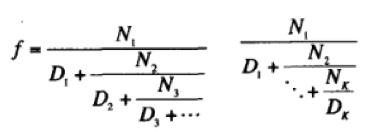``````(define (cont-frac n d k)
;计算 k 项有限连分式：N1 / (D1 + (N2 / (D2 + (N3 / (D3 + ...)))))
(define (cont-inner i)
(cond ((> i k) 0.0)
(else (/ (n k) (+ (d k) (cont-inner (+ i 1)))))))
(cont-inner 1))
(define (cont-frac-iter n d k)
;递归方式计算 k 项有限连分式：从 Nk 和 Dk 的商开始倒推
;依次往前加 d(k-1) 然后被 n(k-1) 除
(define (cont-inner i result)
(if (= i 0) result (cont-inner (- i 1) (/ (n i) (+ (d i) result)))))
(cont-inner (- k 1) (/ (n k) (d k))))
(define (cont-frac-1 k)
;当 Ni 和 Di 都为 1 时，k 项有限连分式会逼近 1/golden-ratio
(cont-frac-iter (lambda (i) 1.0) (lambda (i) 1.0) k))
(printf "~d\n" (cont-frac-1 100))
``````

``````(define (compute-e)
;根据欧拉 De Fractionibus Continuis 文中发现的：Ni 为 1，
;Di 为 1 2 1 1 4 1 1 6 1 1 8 的连分式求 e - 2
(+ 2.0 (cont-frac-iter
(lambda (i) 1.0)
(lambda (i)
(if (= (remainder i 3) 2)
(* 2 (+ 1 (floor (/ i 3))))
1))
1000)))
(printf "e is ~d\n" (compute-e))
``````

``````(define (tan-cf x k)
;基于 Lambert 公式计算正切函数近似值
;tanx = x / (1 - x^2 / (3 - x^2 / (5 - ..)))
(define pow-x (* x x))
(cont-frac-iter (lambda (i) (- (* 2.0 i) 1))
(lambda (i) (if (= i 1) x pow-x))
k))
(printf "tan 30 is ~d" (tan-cf 30 1000))
``````

## 过程作为返回值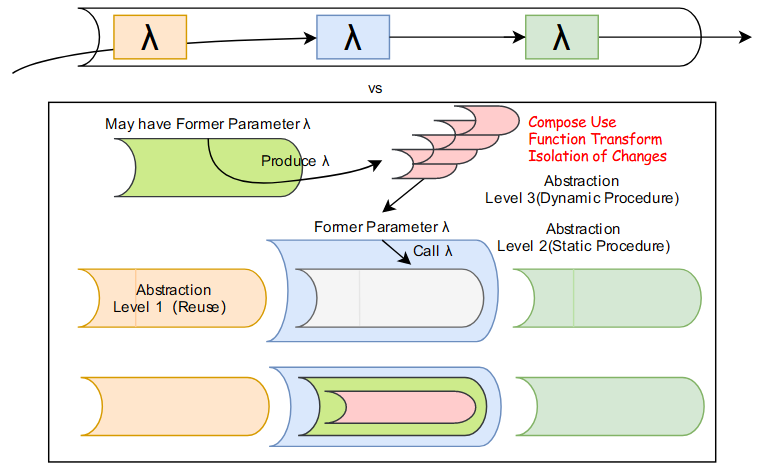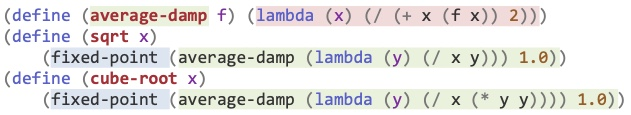``````(define (compose1 f g) (lambda (x) (f (g x))))
(define (compose2 f g x) (f (g x)))
(printf "~d\n" ((compose1 (lambda (x) (* x x)) (lambda (x) (+ x 1))) 6))

(define (repeated1 f n)
(lambda (x)
(define (repeated n)
(if (= n 1) (f x) (f (repeated (- n 1)))))
(repeated n)))
(define (repeated2 f n x)
(if (= n 1) (f x) (f (repeated f (- n 1)))))
(printf "~d\n" ((repeated1 (lambda (x) (* x x)) 2) 5))
``````

smooth 是一种依赖 repeated 的通过多次重复调用 fs 以对函数进行平滑的变换，如下所示，这里的终点在于我们看到了“生产过程”的过程是可以组合和嵌套的：repeated 和 smooth，组合嵌套后仍然保持了原来的抽象。

``````(define (smooth f n)
;对于函数 f(x) 的平滑定义为 f(x-dx), f(x) 和 f(x+dx) 的平均值, dx 为很小值
;本函数可以对 f(x) 进行 n 次平滑
(define dx 0.001)
(define fs (lambda (x) (/ (+ (f (- x dx)) (f x) (f (+ x dx))) 3)))
(define (repeated f n)
(lambda (x)
(define (repeated-inner n)
(if (= n 1) (f x) (f (repeated-inner (- n 1)))))
(repeated-inner n)))
(repeated fs n))
``````

【①过程组合】可能上述应用的“抽象性”体现的不太明显。但下面的例子中，被生成过程的抽象性则体现的淋漓尽致：作为运算符的过程动态生成并叠加：如下所示 double 为将 f 过程应用两次的过程，对于 inc 而言，其递增 2 而非 1，而对于下面这个 (double (double double)) 而言，就是一个持续叠加高阶函数的过程，理解并不复杂，套过程定义直接展开，在这里展开后是： (f (f (f (f (f (f (x))))))，最里面的 f 是 inc，之后依次是 2^0 .. 2^4 是 16，因此最后结果为 5 + 16 = 21。

``````(define (double f)
(lambda (x) (f (f x))))
(printf "~d\n" ((double (lambda (x) (+ x 1))) 1)) ; 3
(printf "~d\n" (((double (double double)) (lambda (x) (+ x 1))) 5)); 21
``````

``````(define (fixed-point f first-guess)
;求解不动点 f(x)=x 方法：不断的将 f(x) 作为 f 的输入，直到得到容忍度内结果
(define tolerance 0.00001)
(define (close? v1 v2) (< (abs (- v1 v2)) tolerance))
(define (try guess step)
(let ((next (f guess)))
(if (close? guess next) next (try next (+ 1 step)))))
(try first-guess 1))
(define (average-damp f) (lambda (x) (/ (+ x (f x)) 2)))
(define (sqrt-1 x) (fixed-point
(average-damp (lambda (y) (- (* y y) x)) 1.0)
1.0))
``````

``````(define (neutron-method g guess)
;牛顿法是一个包含 g 函数的 f 函数:
;f(x)=x-(g(x)/Dg(x)), 其中 Dg(x) 为 g 对 x 的导数
;对此 f 函数求不动点将得到 g(x) = 0 的一个解
(define dx 0.00001)
;导数本质是返回函数的函数，Dg(x)=(g(x+dx) - g(x))/dx, dx 为很小的数
(define (deriv g) (lambda (x) (/ (- (g (+ x dx)) (g x)) dx)))
(define (neutron-transform g)
(lambda (x) (- x (/ (g x) ((deriv g) x)))))
(fixed-point (neutron-transform g) guess))

(define (sqrt-2 x) (neutron-method (lambda (y) (- (* y y) x)) 1.0))
``````

【②函数变换】介于这两种方式之间存在着共性：均基于某种 transform 并应用 fixed-point，因此可以基于“高阶函数作为参数”来抽象，并基于此抽象来解决问题。要注意，实际上刚性的有这种直接需求“动态生产过程”的场景不多 —— 比如这里对过程（函数）应用过程：average-damp 和 neutron-transform，大部分情况下对数据的变换 - Level1，或者需要过程模板普适化，增强适用性 - Level2 更多。

``````(define (fixed-point-of-transform g transform guess)
(fixed-point (transform g) guess))

(define (sqrt-damp x) (fixed-point-of-transform (lambda (y) (/ x y))
average-damp 1.0))

(define (sqrt-newton x) (fixed-point-of-transform (lambda (y) (- (* y y) x))
newton-transform 1.0))
``````

``````(define (fixed-point f first-guess)
;求解不动点 f(x)=x 方法：不断的将 f(x) 作为 f 的输入，直到得到容忍度内结果
(define tolerance 0.00001)
(define (close? v1 v2) (< (abs (- v1 v2)) tolerance))
(define (try guess step)
(printf "step:~d value:~d\n" step guess)
(let ((next (f guess)))
(if (close? guess next) next (try next (+ 1 step)))))
(try first-guess 1))

(define (cube x)
(define (is-good-cube x g)
(< (/ (abs (- x (* g g g))) x) 0.01))
(define (improve-cube x g)
(guess-cube x (/ (+ (/ x (* g g)) (* g 2)) 3)))
(define (guess-cube x g)
(if (is-good-cube x g) g (improve-cube x g)))
(guess-cube x 1.0))

(define (iterative-improve is-good? improve)
(lambda (first-guess)
(define (iter guess)
(define next (improve guess))
(if (is-good? guess next) x (iter next)))
(iter first-guess)))

(define (fixed-point-by-ii f first-guess)
(define tolerance 0.00001)
(iterative-improve
(lambda (guess next) (< (abs (- guess next)) tolerance))
(lambda (guess) (f guess)) first-guess))

(define (cube-by-ii x)
(iterative-improve
(lambda (guess next) (< (/ (abs (- x (* guess guess guess))) x) 0.01))
(lambda (guess) (/ (+ (/ x (* guess guess)) (* guess 2)) 3))
1.0)
``````

【③隔离可变性】这里引出了一个有意思的问题，抽象到 L2：(iterative-improve is-good? improve first-guess) 和 L3：(iterative-improve is-good? improve) (lambda (first-guess) xxx) 的区别是什么？我们现在清楚后者是一种更高级的抽象，其更加困难，而这种困难不是我们人为设置的障碍，而是一种对于“变化”和“不变”进行区分的要求导致的：L3 的抽象可以将一个过程区分为几个不同的抽象层次，就像“过程抽象”将一个过程区分为几个不同的步骤一样。生成过程的过程为我们提供了不同层次的抽象，以便我们更加方便的调用 —— 如果不这样，那么每次依赖最高层抽象时都需要传递大量参数，非常繁琐。比如上面的例子，first-guess 相比较 is-good? 和 improve 这两个参数，很显然变化的概率大得多，因此和这两个过程 is-good? 和 improve 并不应该平级放置，但在 L2 抽象中并没有办法，但在 L3 中，则完全可以将更容易变化的变量作为返回的过程依赖的参数后续提供，这意味着我们提供了一种像洋葱一样的模型，核心是最不容易变化的部分，而从核心到外围，每一层都生产新的过程，每个过程都依赖更容易变化的变量，这种模型可以很好的应对代码变动、修改对核心功能造成的影响，以保证核心代码的可靠性，是一种开发的最佳实践。